# Mapping in R using the `ggplot2` package

One of my favorite packages for creating maps in R is `ggplot2`. No matter what, though, creating maps in R is trickier than doing it in a GIS system, particularly when you don't have 'on the fly' projection as you have in both ArcGIS and QGIS. To help you create maps on your own we share a typical use case below (including a coordinate system mismatch). In this example we use points and polygons by themselves but if you'd like to include tilemaps from Google, Stamen and others you should check out the `ggmap` package.

### 1. Read in the point and polygon data

Start by reading in the data. Our point data is in a comma-separated file with latitude and longitude values. Our polygons are a shapefile of NYC counties (also known as boroughs). You can get a similar file from the NYC Department of City Planning. Note that we're using the `readOGR` function from the package `rgdal` instead of `readShapePoly` from `maptools`. You'll see why later in the post.

``````  library(rgdal)
library(ggplot2)
# read in point data (tabular data)
##       id buildarea latitude longitude
## 1  10023    12.820    40.76    -73.99
## 2  10027    22.092    40.76    -73.99
## 3 10030A    26.081    40.76    -73.98
## 4 10030B    25.597    40.75    -73.98
## 5  10072     3.775    40.76    -73.82
## 6  10225    16.416    40.76    -73.97

# the shapefile of NYC counties/boroughs. Careful about how you define the path
# and layer. I always find this odd.
## OGR data source with driver: ESRI Shapefile
## Source: "nybb.shp", layer: "nybb"
## with 5 features and 6 fields
## Feature type: wkbMultiPolygon with 2 dimensions
``````

### 2. Make some simple maps using `ggplot()`

Now we can create the maps in the same way we make non-geographic charts in `ggplot`. (If you know NYC, you know that the map is distorted — don’t worry we will fix this in the last step).

``````  # map the counties
ggplot() +  geom_polygon(data=counties, aes(x=long, y=lat, group=group))
``````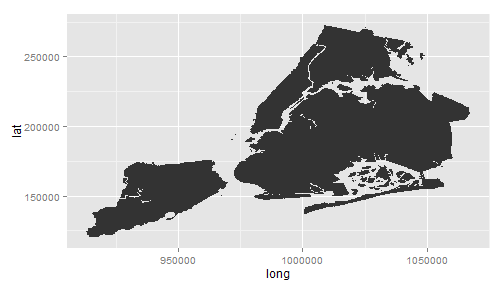``````  # map the points
ggplot() +  geom_point(data=mapdata, aes(x=longitude, y=latitude), color="red")
``````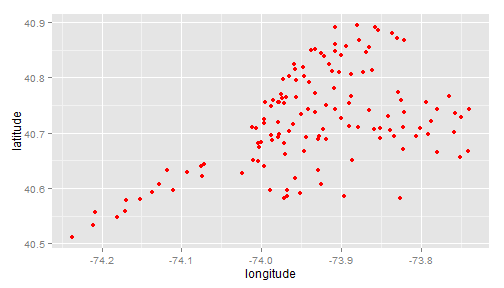Great, this is easy. Let's combine the two:

``````  # map both polys and points together
ggplot()+geom_polygon(data=counties, aes(x=long, y=lat, group=group)) +
geom_point(data=mapdata, aes(x=longitude, y=latitude), color="red")
``````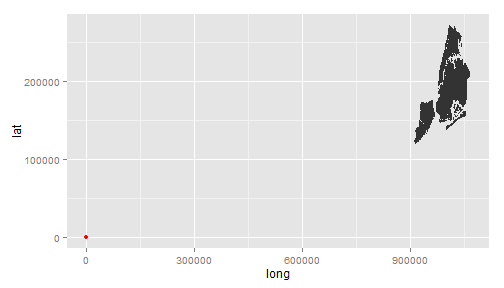Shucks! Usually when you see something odd like this it's related to inconsisitent projections.

### 3. Make the projections consistent

Projecting geographic data is a pain no matter how long you've been doing it. Even though I've been mapping with R for many years I still need to refer to my cheat sheet with some common projections and examples of the code needed to project.

[Note that `ggplot2` does have a function called `coord_map()` that can be used as a sort of on-the-fly projection but the function is still experimental so we’re sticking with this approach for now.]

Before projecting we need to know the existing projection/coordinate system for the layers. For the counties we can use the function `proj4string`. Note, though, that if you read in the shapefile using `readShapePoly` from the library `maptools` instead of `readOGR` the projection information will not be included.

``````proj4string(counties)
##  "+proj=lcc +lat_1=40.66666666666666 +lat_2=41.03333333333333 +lat_0=40.16666666666666 +lon_0=-74 +x_0=300000 +y_0=0 +datum=NAD83 +units=us-ft +no_defs +ellps=GRS80 +towgs84=0,0,0"
``````

Well, the proj4 format is about as clear as mud. To get a more readable format you can look at the .prj file that came with shapefile. For this one you would see the following (note that what you’ll actually see is plain text — the nice formatting you see here comes from spatialreference.org):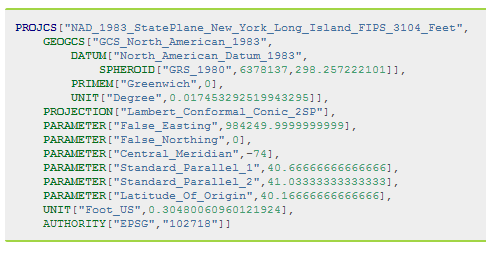This is a good, common projection for New York City – Long Island State Plane (NAD83). But looking at the tabular point data file I see latitude and longitude coordinates (not X/Y) so I know that the point data is not projected. I need to project the point data to State Plane to match the polygon data. To do this we use the `spTransform()` function (from the `rgdal` package). But in order to use it we need to convert the points data frame to class `SpatialPointsDataFrame`.

``````class(mapdata)
##  "data.frame"
coordinates(mapdata)<-~longitude+latitude
class(mapdata)
##  "SpatialPointsDataFrame"
## attr(,"package")
##  "sp"

# does it have a projection/coordinate system assigned?
proj4string(mapdata) # nope
##  NA

# we know that the coordinate system is NAD83 so we can manually
# tell R what the coordinate system is

# now we can use the spTransform function to project. We will project
# the mapdata and for coordinate reference system (CRS) we will
# assign the projection from counties

mapdata<-spTransform(mapdata, CRS(proj4string(counties)))

# double check that they match
identical(proj4string(mapdata),proj4string(counties))
##  TRUE
``````

### 4. Back to the maps

``````
# ggplot can't deal with a SpatialPointsDataFrame so we can convert back to a data.frame
mapdata<-data.frame(mapdata)

# we're not dealing with lat/long but with x/y
# this is not necessary but for clarity change variable names
names(mapdata)[names(mapdata)=="longitude"]<-"x"
names(mapdata)[names(mapdata)=="latitude"]<-"y"

# now create the map
ggplot() +geom_polygon(data=counties, aes(x=long, y=lat, group=group))+  geom_point(data=mapdata, aes(x=x, y=y), color="red")
``````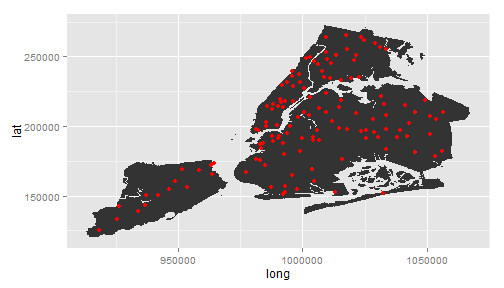Ahh, that’s better. Still not pretty, but we have the pieces working.

### 5. Final touches

Here we're going make the map a little nicer. Get rid of axes, new color gradient, clean up title etc.

``````ggplot() +
geom_polygon(data=counties, aes(x=long, y=lat, group=group), fill="grey40",
colour="grey90", alpha=1)+
labs(x="", y="", title="Building Area Within 1000m")+ #labels
theme(axis.ticks.y = element_blank(),axis.text.y = element_blank(), # get rid of x ticks/text
axis.ticks.x = element_blank(),axis.text.x = element_blank(), # get rid of y ticks/text
plot.title = element_text(lineheight=.8, face="bold", vjust=1))+ # make title bold and add space
geom_point(aes(x=x, y=y, color=buildarea), data=mapdata, alpha=1, size=3, color="grey20")+# to get outline
geom_point(aes(x=x, y=y, color=buildarea), data=mapdata, alpha=1, size=2)+
colours=c( "#f9f3c2","#660000"))+ # change color scale
coord_equal(ratio=1) # square plot to avoid the distortion
``````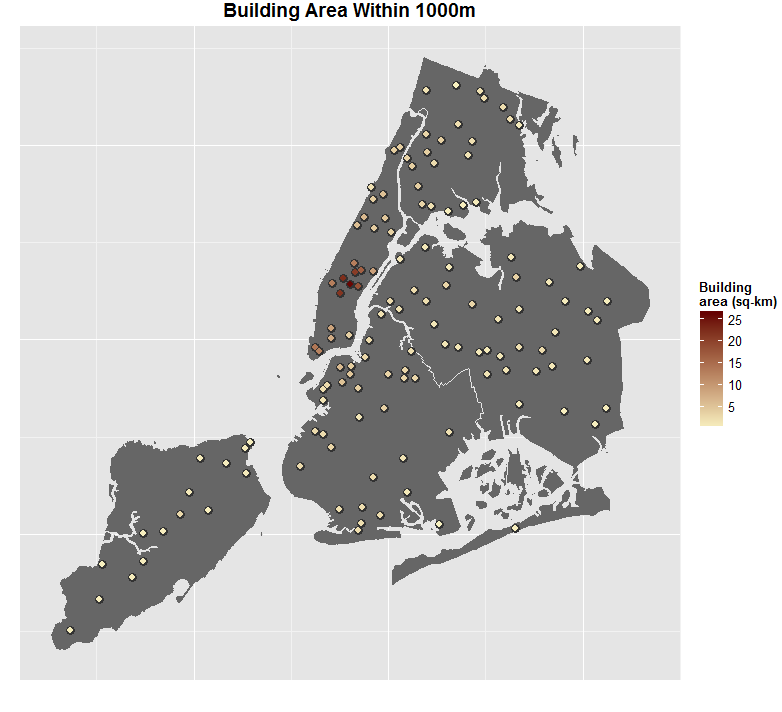## 26 responses

1.Adam says:

Just wanted to say thank you for a wonderful write up. Very clear and understandable for someone who is new to spatial data in R.

•zev@zevross.com says:

Thanks!

2.Mateusz says:

This is great! I’d been struggling with a conversion of a GeoTiff file to a lon lat coordinate system to overplot with data and ultimately I gave up; the bar is high on this stuff, especially to non-geographers. It seems that your walkthrough will guide me through exactly what I need to do. Your writeup is super-clear and understandable and contains a real-life example — just what some of the R documentation is badly in need of. Well done & thanks.

•zev@zevross.com says:

Thanks! Did you end up solving your issue? The `raster` package is really great for this — `projectRaster()` might be what you need. But if possible you’d likely want to make a map with a projected (rather than geographic) coordinate system. Good luck.

3.Flore says:

Clear and easy. Thanks !

4.Shreekant Deodhar says:

This has been of TREMENDOUS help. Thank you so much!! 🙂

5.Ajilesh says:

I got an error saying “Error in eval(expr, envir, enclos) : object ‘buildarea’ not found”

•Ajilesh says:

Sorry. I got it fixed. Thank You for this nice post.

6.anne says:

thanks for posting this – very helpful!

7.Helen says:

8.Muki says:

Great post.. much appreciated. Is there a way to only plot Manhattan and Brooklyn as opposed to plotting all five (5) boroughs.

•zev@zevross.com says:

Sure. You’d filter the county polygons to Manhattan and use this in place of polygons. Then you could use the over function from sp to identify the points that land in Manhattan and re-plot.

9.Adolfo says:

Hi, I found your post by googling how to translate geographic coordinates to NYC borough names. The thing is, I have a big dataset of points in NYC and I want to assign each coordinate to his correspondent borough. I just want a new dataset with an extra column with the borough name, is this possible by using the packages that you used in this post? I already have a shapefile from NYC Boroughs. Thanks and great post!

•zev@zevross.com says:

Check out the over function, you need to do a little work, but that should help.

•Adolfo says:

The over function in which package? Thanks!

•zev@zevross.com says:

From the sp package

•zev@zevross.com says:

Yes I use ggmap often and mean I write a post about it but haven’t, thanks for the note!

10.Hayley Rogers says:

Hello,

I am getting an error when I try to remove ticks and text from my ggplot axis… It says “Error: Don’t know how to add RHS to a theme object” –> is there a special packages to use theme?

•Rafa Pereira says:
•zev@zevross.com says:

Thanks Rafa

11.Abhay says:

Hi,

Its great tutorial.

Btw could we please tell me how to add label/city names/country names on the above map?

Thanks

12.Nathan says:

Hello. I would like to replicate this as a learning exercise, but I am not sure where the “mapdata.csv” file comes from. What file are you using for your tabular data?

•zev@zevross.com says:

If you send me an e-mail I’ll send it to you, sorry for the delay.

13.Andrea says:

Great post! However, when I tried to assign de CRS with the line below,

proj4string(correMDP)<-CRS("+proj=longlat + datum=WGS84")

an error showed up:

Error in `proj4string<-`(`*tmp*`, value = new("CRS", projargs = "+proj=longlat +ellps=WGS84")) :
Geographical CRS given to non-conformant data: 843581 10087897

Do you have any idea of how to fix it?

•zev@zevross.com says:

You should take a look at your coordinate data. Does it look like latitudes and longitudes? Or are they big numbers (suggesting that they are X, Y). The coordinate system WGS84 is non-projected so it expects lats and longs.